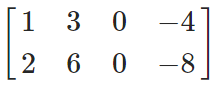# Describe all solutions of Ax=0 in parametric vector form, where A is row equivalent

Describe all solutions of Ax=0 in parametric vector form, where A is row equivalent to the given matrix.